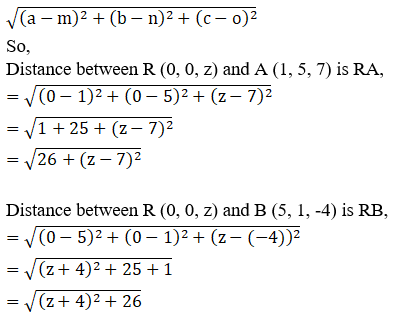Determine the point on z-axis which is equidistant from the points (1, 5, 7) and (5, 1, -4)

Asked by Sakshi | 1 year ago |  38

##### Solution :-

Given:

The points (1, 5, 7) and (5, 1, -4)

We know x = 0 and y = 0 on z-axis

Let R(0, 0, z) any point on z-axis

According to the question:

RA = RB

RA2 = RB2

By using the formula,

The distance between any two points (a, b, c) and (m, n, o) is given by,We know, RA2 = RB2

26+ (z – 7)2 = (z + 4)2 + 26

z2+ 49 – 14z + 26

= z2+ 16 + 8z + 26

49 – 14z = 16 + 8z

49 – 16 = 14z + 8z

22z = 33

z =$$\dfrac{33}{22}$$

$$\dfrac{3}{2}$$

The point R (0, 0,$$\dfrac{3}{2}$$) on z-axis is equidistant from (1, 5, 7) and (5, 1, -4).

Answered by Aaryan | 1 year ago

### Related Questions

#### A(1, 2, 3), B(0, 4, 1), C(-1, -1, -3) are the vertices of a triangle ABC. Find the point in which the bisector of the

A(1, 2, 3), B(0, 4, 1), C(-1, -1, -3) are the vertices of a triangle ABC. Find the point in which the bisector of the angle ∠BAC meets BC.

#### The mid-points of the sides of a triangle ABC are given by (-2, 3, 5), (4, -1, 7) and (6, 5, 3). Find the coordinates

The mid-points of the sides of a triangle ABC are given by (-2, 3, 5), (4, -1, 7) and (6, 5, 3). Find the coordinates of A, B and C.

#### If the points A(3, 2, -4), B(9, 8, -10) and C(5, 4, -6) are collinear, find the ratio in which C divided AB.

If the points A(3, 2, -4), B(9, 8, -10) and C(5, 4, -6) are collinear, find the ratio in which C divided AB.